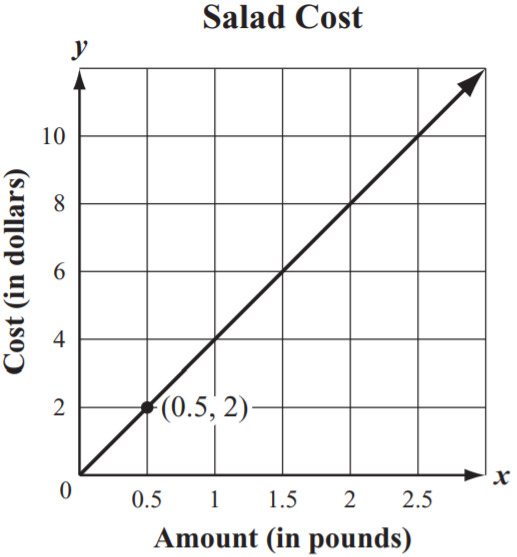PSA79MT - Grade 8 Mathematics Practice Test 2014

What is the value of the expression below?Select one:

Frank wrote his initial to the left of a line, as shown below.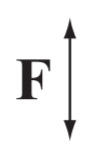Then he reflected his initial over the line. Which of the following shows Frank’s initial after the reflection?

A)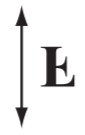B)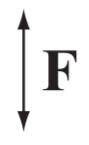C)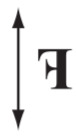D)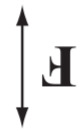Select one:

The volume of a cube is 64 cubic inches. What is the length of one edge of the cube?

Select one:

Which ordered pair is the solution of the system of equations below?

x+2y=6

3x+8y=4

Select one:

Juan purchased one binder for \$3.50 and f folders for \$1.25 each. The total cost, C, in dollars, of Juan’s purchase is represented by the equation below.

C=3.50+1.25f

Which of the following graphs represents C, the total cost of Juan’s purchase if he buys different numbers of folders?

A)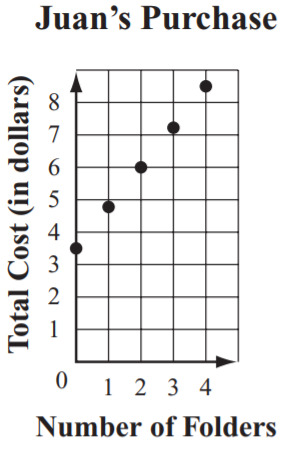B)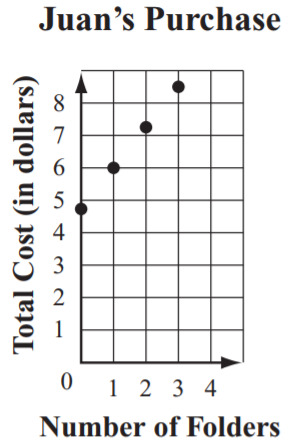C)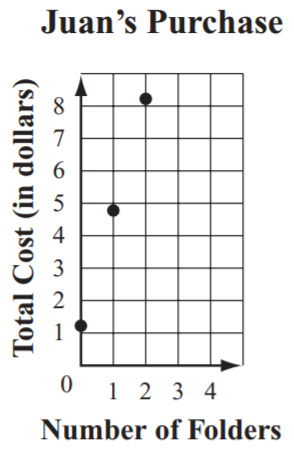D)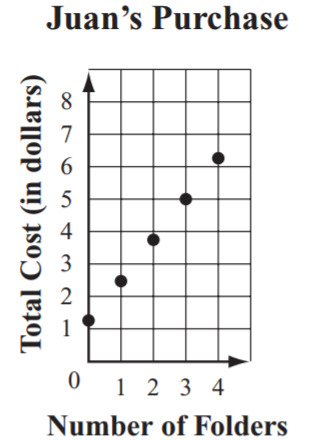Select one:

List the following expressions in order from least to greatest value.

Type the correct order of each item (eg. 1, 2, 3, etc):

The average distance between planet Neptune and the Sun is about 4×10^9 kilometers. What is 4×10^9 written in standard notation?

Select one:

Triangle PQR, triangle RST, and two angle measures are shown below.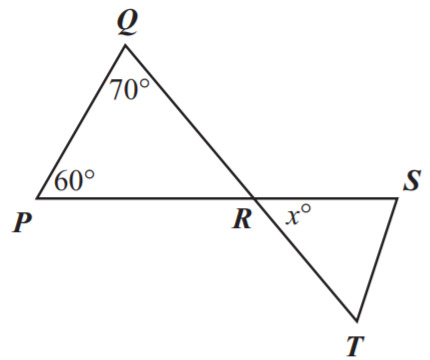Line segment QT intersects line segment PS at point R. What is the value of x? Do not include units (°) in your answer.

Type your answer below as a number (example: 5, 3.1, 4 1/2, or 3/2):

Which of the following equations represents a linear function?

Select one:

What is the value of the expression below?Select one:
Part A)

A principal surveyed 75 seventh-grade and eighth-grade students. She asked them if they prefer to obtain the news from the Internet or to obtain the news from television. She created a table to display the data, as shown below.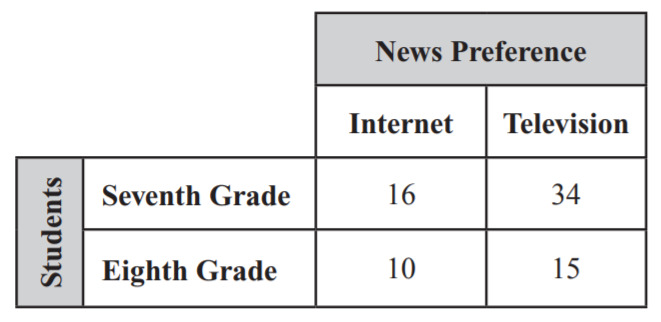a) How many seventh-grade students responded to the survey?

Type your answer below as a number (example: 5, 3.1, 4 1/2, or 3/2):
Part B)

Part C)

b) What is the relative frequency of seventh-grade students who prefer to obtain the news from the Internet to all the seventh-grade students surveyed?

Type your answer below as a number (example: 5, 3.1, 4 1/2, or 3/2):
Part D)

Part E)

c) What is the relative frequency of eighth-grade students who prefer to obtain the news from the Internet to all the eighth-grade students surveyed?

Type your answer below as a number (example: 5, 3.1, 4 1/2, or 3/2):
Part F)

Part G)

d) Is there evidence in the principal’s survey that eighth-grade students prefer to obtain the news from the Internet more than seventh-grade students do?

Select one:
Part H)

The graph below shows the temperature, in degrees Fahrenheit, of a liquid for the first ten minutes of a heating experiment.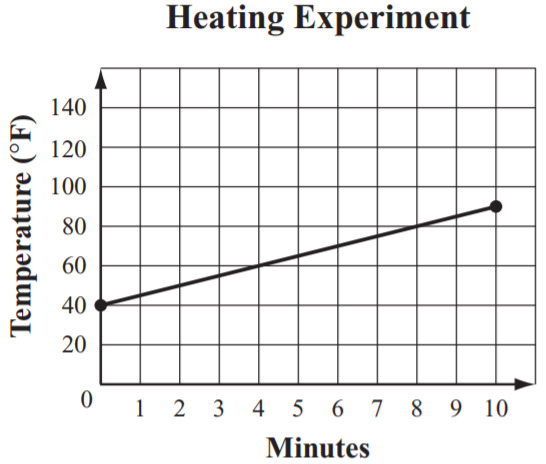Based on the graph, which expression could be used to calculate the temperature of the liquid after m minutes?

Select one:

Ted has some red blocks and some green blocks.

• Each red block weighs the same number of ounces.

• Each green block weighs the same number of ounces.

• The total weight of 2 red blocks and 6 green blocks is 23 ounces.

• The total weight of 3 red blocks and 4 green blocks is 22 ounces.

What is the total weight of 1 red block and 1 green block?

Select one:

A stained glass window is in the shape of a square. A sketch of the window, with some of its dimensions, is shown below.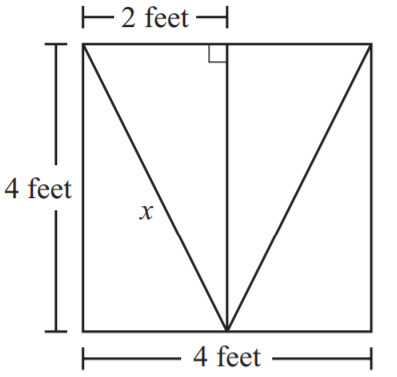What is the length, to the nearest tenth of a foot, of the line segment labeled x?

Select one:

The graph below shows the relationship between the distance a delivery truck is driven and the amount of gas the truck uses.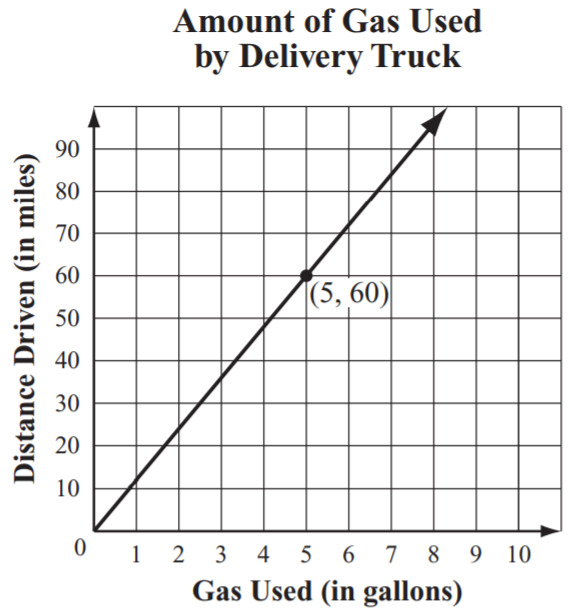Based on the graph, what is the average distance, in miles, the truck can be driven using 1 gallon of gas?

Select one:

Kiki is taking a bicycle ride. During the ride, Kiki is always traveling away from the starting point. Which of the following graphs of distance and time could model Kiki’s ride?

A)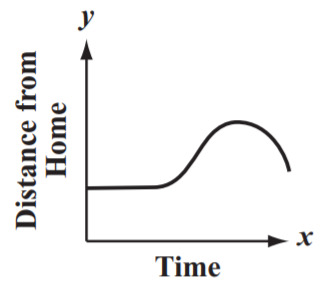B)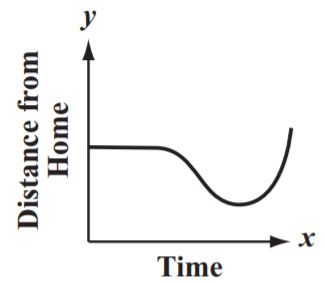C)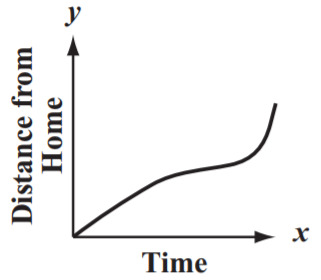D)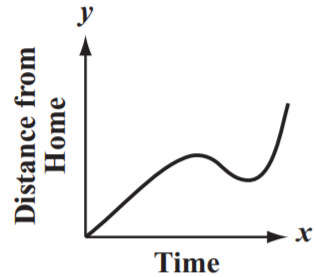Select one:

3(2-k)=-3k+2

Which statement is true about the equation below?

Select one:

Which of the following graphs shows a linear function?

A)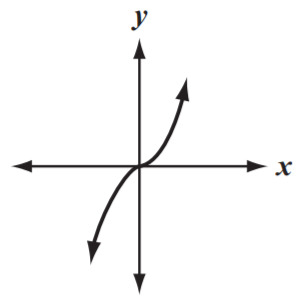B)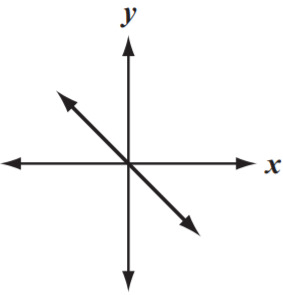C)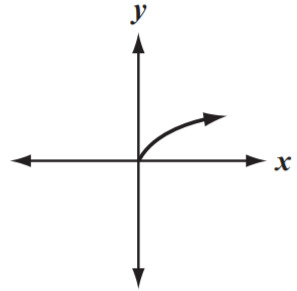D)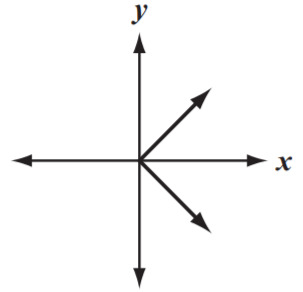Select one:

The table below shows a relationship between x and y that is not a function.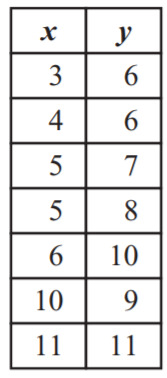Write one ordered pair that can be removed from the table to make the relationship between x and y a function.

(x,y)

with no spaces.

Part A)

Pentagon PQRST is shown on the coordinate grid below.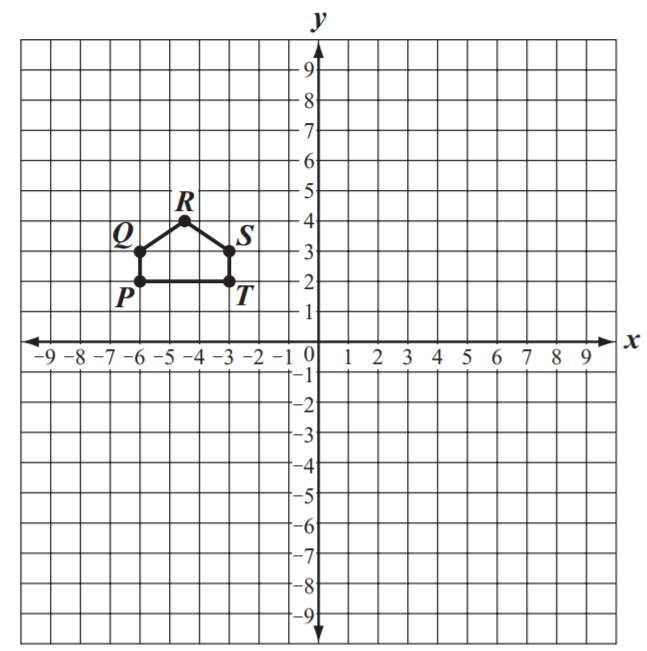a) What are the coordinates for vertex T?

(x,y)

with no spaces.

Part B)

b) Draw the image of pentagon PQRST after it has been translated 6 units to the right. Label it P'Q'R'S'T'. Be sure to copy the original pentagon PQRST on your graph as well.

Draw your graph on paper, take a picture, and upload it using the image upload icon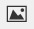If you do not have the ability to upload an image of your work type "Graph is on paper."

Part C)

c) What are the coordinates of vertex T'?

(x,y)

with no spaces.

Part D)

d) Draw the image of pentagon P'Q'R'S'T' after it has been rotated 180° clockwise about vertex T'

Draw your graph on paper, take a picture, and upload it using the image upload iconIf you do not have the ability to upload an image of your work type "Graph is on paper."